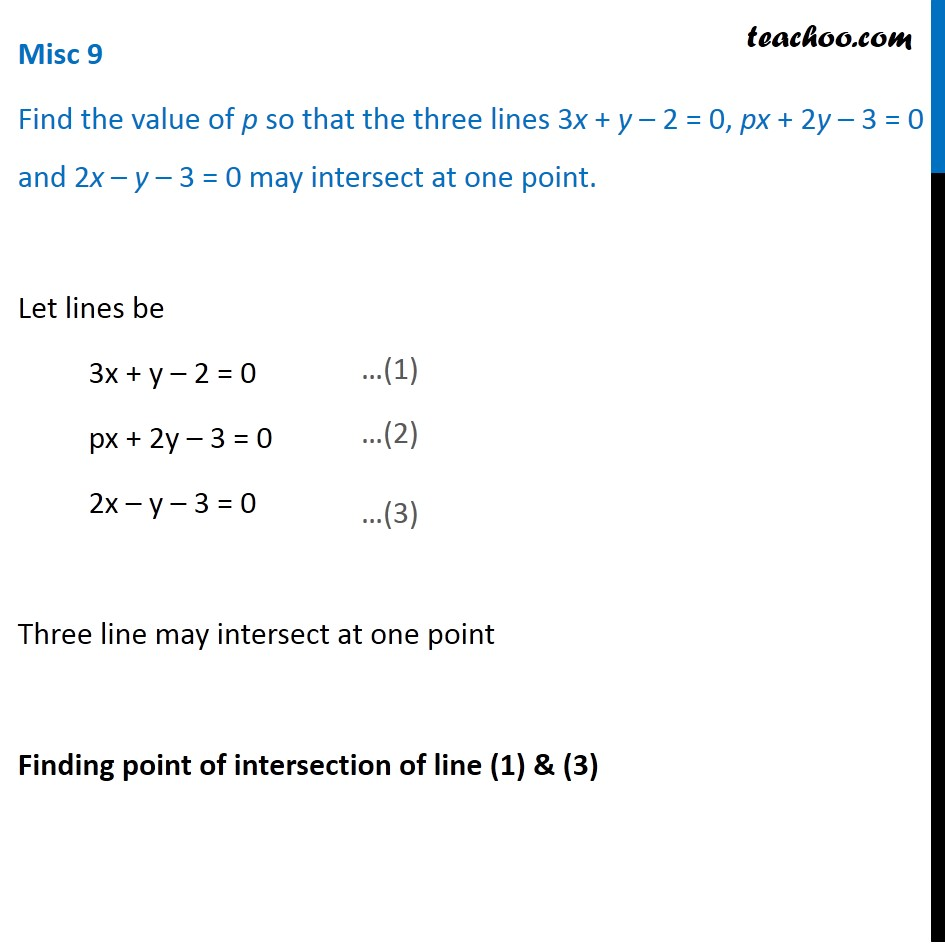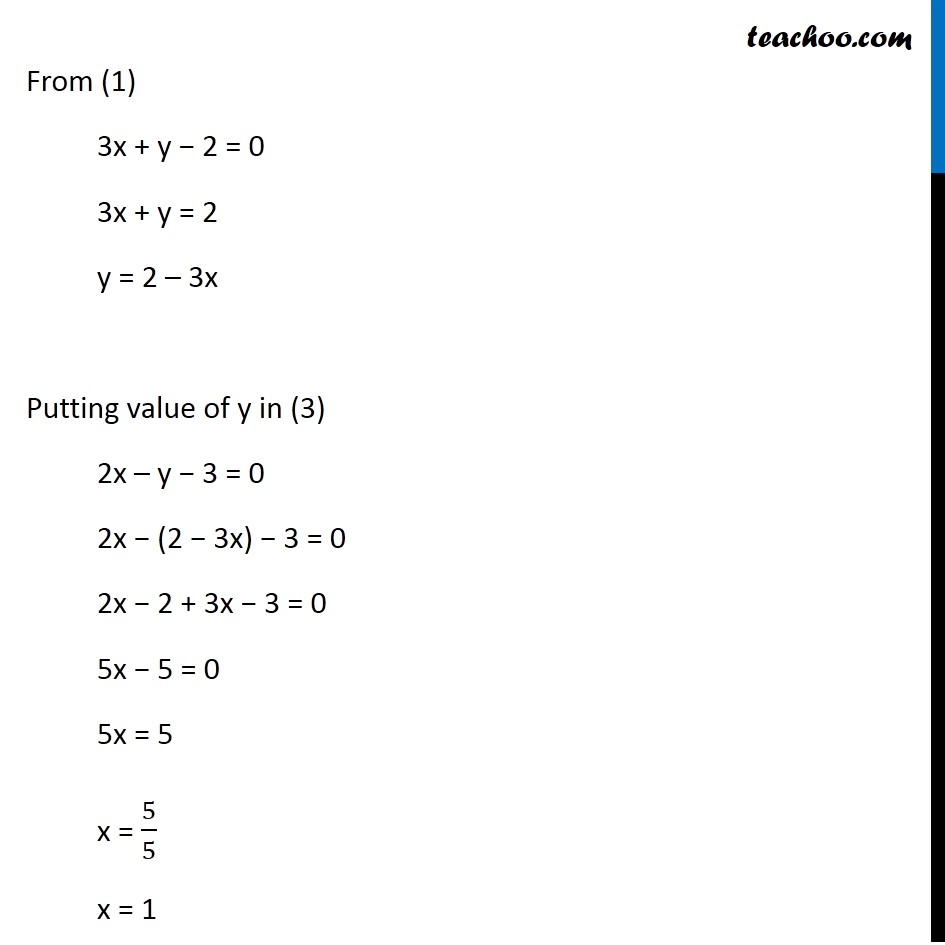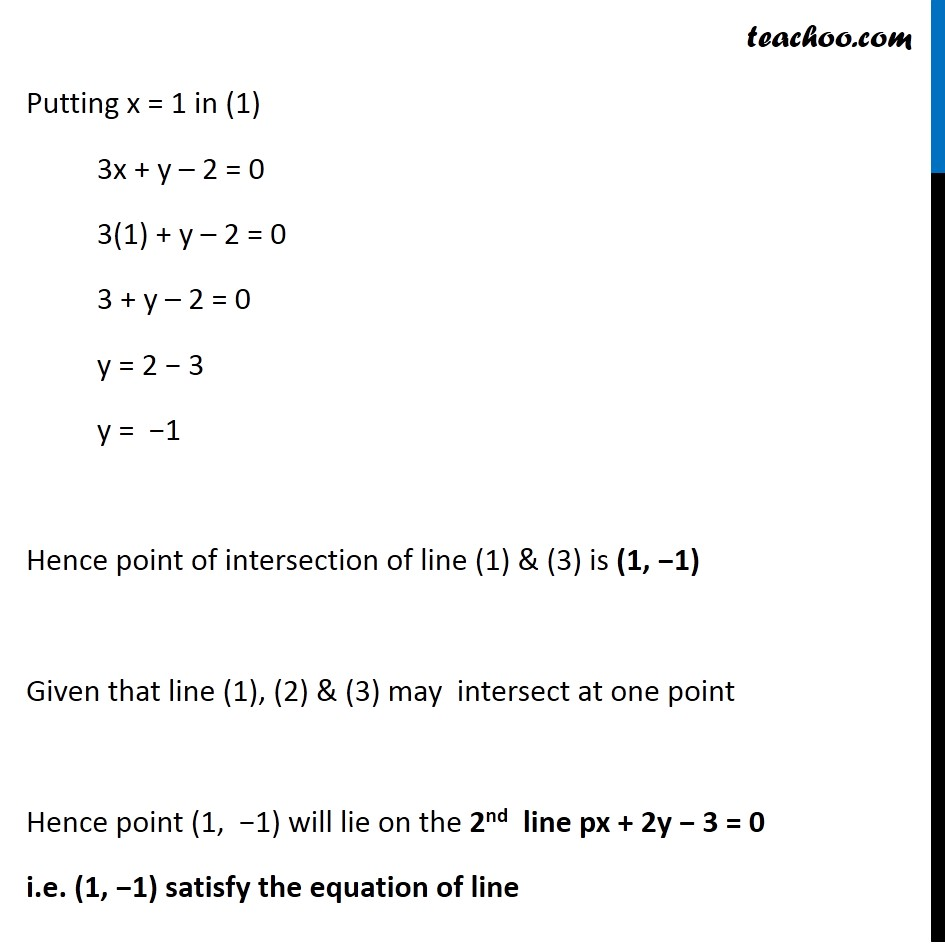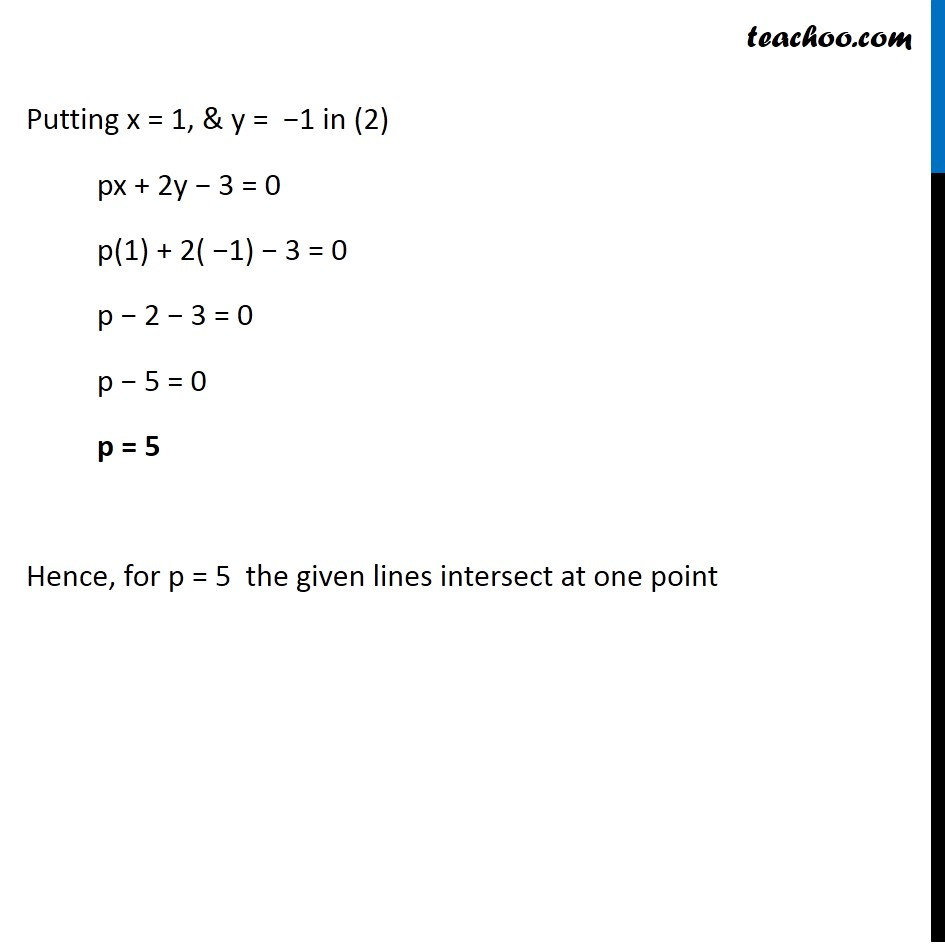Miscellaneous

Chapter 9 Class 11 Straight Lines
Serial order wiseLearn in your speed, with individual attention - Teachoo Maths 1-on-1 Class

### Transcript

Misc 8 Find the value of p so that the three lines 3x + y – 2 = 0, px + 2y – 3 = 0 and 2x – y – 3 = 0 may intersect at one point. Let lines be 3x + y – 2 = 0 px + 2y – 3 = 0 2x – y – 3 = 0 Three line may intersect at one point Finding point of intersection of line (1) & (3) From (1) 3x + y − 2 = 0 3x + y = 2 y = 2 – 3x Putting value of y in (3) 2x – y − 3 = 0 2x − (2 − 3x) − 3 = 0 2x − 2 + 3x − 3 = 0 5x − 5 = 0 5x = 5 x = 5/5 x = 1 Putting x = 1 in (1) 3x + y – 2 = 0 3(1) + y – 2 = 0 3 + y – 2 = 0 y = 2 − 3 y = −1 Hence point of intersection of line (1) & (3) is (1, −1) Given that line (1), (2) & (3) may intersect at one point Hence point (1, −1) will lie on the 2nd line px + 2y − 3 = 0 i.e. (1, −1) satisfy the equation of line Putting x = 1, & y = −1 in (2) px + 2y − 3 = 0 p(1) + 2( −1) − 3 = 0 p − 2 − 3 = 0 p − 5 = 0 p = 5 Hence, for p = 5 the given lines intersect at one point